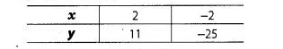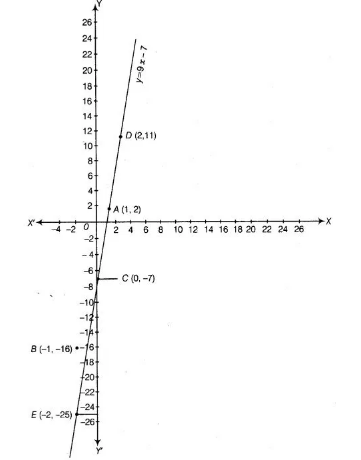# Show that the points A (1, 2), B (-1, -16)`
Question:

Show that the points A (1, 2), B (-1, -16) and C (0, -7) lie on the graph of the linear equation y = 9x – 7.

Thinking Process

(i) Firstly, make a table for the equation y = 9x – 7.

(ii) Secondly, plot the obtained points from the table on a graph and join them to get a straight line.

(iii) Further, we plot the given points on a graph paper and find that whether these points lie on the line or not.

Solution:

Firstly, to draw the graph of equation

$y=9 x-7$

When $x=2$, then $y=9 \times 2-7$

$=18-7=11$

When $x=-2$, then $y=9 \times-2-7$

$=-18-7=-25$Here, we find two points $D(2,11)$ and $E(-2,-25)$.

So, draw the graph by plotting the points and joining the line $D E$.Now, we plot the given points A (1,2), B (-1, -16) and C (0, -7) on the graph paper. We see that all the points lie on DE line.